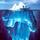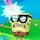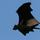## General QuestionAsked by gasman (11315) September 12th, 2011

I’ve worked with MS Office Excel spreadsheets before. I understand referencing other cells in a formula, specifying a range of cells, and using functions like SUM(ABOVE) OR SUM(LEFT). But I’m stumped on how to implement a couple of seemingly simple situations:

(1) Make a table where the first column consists of positive numbers and the second column is their square roots. Each cell in the 2nd column needs a formula that says, “Take the square root of the value in the cell to your left.” How would you write the formula? Do you have to enter a different formula for each row?

(2) Make a multiplication table for the integers 1–9 (like in 3rd grade) where the top row and leftmost column each contain values 1 through 9. Cell i9 would contain the number 81. How do you write a formula that says, “Take the first number in this row (i.e., the value of the first cell) & multiply by the first number in this column?”

Observing members: 0Composing members: 0Which version of excel are you using? In 2010 it has a square root function which is =sqrt(CellNameHere). Also as far as the second problem with the multiplication table can you not just use the cell name for example =\$B\$1*A2 and then drag it down?

abysmalbeauty (2734)“Great Answer” (2) Flag as…@abysmalbeauty Not sure what you mean by “drag it down.”

gasman (11315)“Great Answer” (1) Flag as…In column A:A you’ll have a series of values.

1)
The formula in B1 is: =sqrt( A1)
Select and copy that cell down the full column (as far down as you have values in A:A), and the formula for B2 will show: =sqrt( A2) and so on.

2)
Set A2:A10 as values 1 – 9
Set B1:J1 as values 1 – 9

At B2 the formula is (exactly this): =B\$1 * \$A2

Copy that cell

Select cells B2:J10 and paste the copied formula. (The ending formula at J10 will be: =J\$1 * \$A10)

I hope this wasn’t homework in Excel.

CWOTUS (26082)“Great Answer” (7) Flag as…tutorial

Check out that website for a tutorial on autofilling a formula

abysmalbeauty (2734)“Great Answer” (6) Flag as…@CWOTUS and @abysmalbeauty: The automatic fill feature seems to be the answer I’m looking for. Who knew? Thanks a million.

Homework? Ha! Not since Nixon was president…

That’s a great tutorial, @abysmalbeauty. Cuts through the usual b.s.

gasman (11315)“Great Answer” (3) Flag as…Happy to help :)

again dependent on what version of excel you are using however if you are using a newer version, double clicking on the teeny tiny square at the bottom of the cell that is used to auto fill will automatically drag it down to the bottom of your table for you.

abysmalbeauty (2734)“Great Answer” (0) Flag as…Actually I just installed the latest Office for Mac (previously used Office 2003 for Windows). This feature might have been there all along, but I didn’t know about it until I “tapped the collective” yesterday.

gasman (11315)“Great Answer” (0) Flag as…or